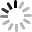# Google 試算表 (2) - 讀取儲存格數值

## 讀取儲存格數值``````function doGet(e) {
var url = '試算表網址';
var name = '工作表1'
}
````````````getSheetValues(startRow, startColumn, numRows, numColumns)
``````

``````getSheetValues(startRow, startColumn, 1,1)
``````

``````function doGet(e) {
var url = '試算表網址';
var name = '工作表1'
Logger.log(SheetName.getSheetValues(1,1,1,1));   // 取得 A1 數值
Logger.log(SheetName.getSheetValues(4,2,1,1));   // 取得 B4 數值
}
````````````function doGet(e) {
var url = '試算表網址';
var name = '工作表1'
Logger.log(SheetName.getSheetValues(1,1,4,4));
}
``````## getLastColumn()、getLastRow()

``````function doGet(e) {
var url = '試算表網址';
var name = '工作表1'
var lastColumn = SheetName.getLastColumn();
var lastRow = SheetName.getLastRow();
Logger.log(SheetName.getSheetValues(1,1,lastRow,lastColumn));
}
``````# 小結

``````function doGet(e) {
var url = '試算表網址';
var name = '工作表1'
var lastColumn = SheetName.getLastColumn();
var lastRow = SheetName.getLastRow();
Logger.log(SheetName.getSheetValues(1,1,lastRow,lastColumn));
Logger.log(SheetName.getColumnWidth(1));  // 抓取 A 的寬度
Logger.log(SheetName.getRowHeight(1));    // 抓取第一列高度
Logger.log(SheetName.getTabColor());      // 抓取 tab 顏色，沒有指定顏色就會顯示 null
}
``````